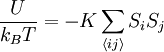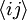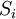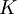Ising model

The Ising model is also known as the Lenz-Ising model. For a history of the Lenz-Ising model see Refs. 1 and 2. The Ising model is commonly defined over an ordered lattice. Each site of the lattice can adopt two states: either UP (S=+1) or DOWN (S=-1).

The energy of the system is the sum of pair interactions between nearest neighbors.$\frac{U}{k_B T} = - K \sum_{\langle ij \rangle} S_i S_j$

where$k_B$ is the Boltzmann constant,$T$ is the temperature,$\langle ij \rangle$ indicates that the sum is performed over nearest neighbors, and$S_i$ indicates the state of the i-th site, and$K$ is the coupling constant.

2-dimensional Ising model

Solved by Lars Onsager in 1944. Rudolf Peierls had previously shown (1935) that, contrary to the one-dimensional case, the two-dimensional model must have a phase transition.

3-dimensional Ising model

Sorin Istrail has shown that the solution of Ising's model cannot be extended into three dimensions for any lattice:

ANNNI model

The axial next-nearest neighbour Ising (ANNNI) model is used to study alloys, adsorbates, ferroelectrics, magnetic systems, and polytypes.